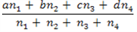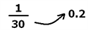# Weighted Average

Weighted average is a mean calculation that takes into account the variable degrees of importance of the numbers in a data set. It is an important section in CAT Quantitative Aptitude. Weighted average is an average in which each quantity to be averaged is assigned a weight, and these weightings determine the relative importance of each quantity on the average. Weightings are the equivalent of having that many similar items with the same value involved in the average.

## Weighted Average Formula

Observe that the average can be calculated directly only if the weights of all the factors are the same. If the values a, b, c and d are the individual averages of each of the groups, and n1, n2, n3 and n4 are the numbers of observations or numbers of values, then the weighted average is calculated by the Weighted Average Formula given below:The term “weight” stands for the relative importance attached to the different values.

1) In Class I, there are 12 students with an average age of 20 years, and in Class II, there are 16 students with an average age of 23 years. What is the average age of both classes?

Solution:

=((12 ×20)+(16 ×23))/((12+16)) = (240+368)/28 = 608/28 = 20.7 years

Note:

➜ Average always lies between the maximum and minimum values. It will be equal to the maximum or minimum value if all the values are equal

➜ Average is the result of the net surplus + net deficit

➜ If the value of each quantity is increased or decreased by the same quantity “q”, then the average will also increase or decrease by the quantity “q” respectively.

➜ If the value of each quantity is multiplied or divided by a quantity “q”, then the resultant average will also be multiplied or divided by “q” respectively.

➜ When the weights of all the quantities are the same, then the average can be calculated directly. If the weights are different, we need to use the concept of weighted average.

## Short-cut Techniques in Weighted Average

Let us now see a useful shortcut technique to find the weighted average with the help of an example.

2) The average of a batsman in 16 innings is 36. In the next innings, he scored 70 runs. What will be his new average?

1. a) 44 b) 38 c) 40 d) 48

Solution:

#### Conventionally solving

New average = (old sum+ new score)/(total number of innings) = ((16 ×36)+70)/((16+1)) = 38

#### Shortcut technique:

Step 1: Take the difference between the new score and the old average = 70-36 = 34

Step 2: This 34 extra run is spread over 17 innings = 34/17 = 2

Step 3: Hence, the average increases by 2 = >36+2 = 38 is the new average

Let us try another question using the same technique.

3) The average mark of 19 children in a particular school is 50. When a new student with marks of 75 joins the class, what will be the new average of the class?

Solution:

1) Take the difference between the old average and the new marks = 75-50 = 25

2) This score of 25 is distributed over 20 students => 25/20 = 1.25

3) Hence, the average increases by 1.25=> 50+1.25 = 51.25

Let us try another question where the average dips.

4) The average age of Mr. Mark’s 3 children is 8 years. A new baby is born. Find the average age of all his children.

Solution:

The new age will be 0 years. The difference between the old average and the new age = 0-8 = -8

This age of 8 years is spread over 4 children = > (-8/4 = -2) Hence, the average reduces to 8-2 = 6 years

Now, let us look at a technique that will help us compute the new value when the average is given.

Take this question, for example.

5) The average age of 29 students is 18. If the age of the teacher is also included, the average age of the class becomes 18.2. Find the age of the teacher.

1. a) 28 b) 32 c) 22 d) 24

Solution:

#### Conventionally solving

Let the average age of the teacher =x

(29 × 18 + x × 1)/30

Solving for x, we get x = 24

Using the shortcut based on the same method we used previously,

Step 1: Calculate the change in average = 18.2-18 = 0.2

This change in 0.2 is reflected over a sample size of 30Therefore, the teacher’s age is an increase by 30 * 0.2 = 6 years above the average, 18+6 = 24.

Think Question – The average age of 26 students in an MBA school is 30. One student among these quits school in between. Can you find the age of that student if the new average is 29.8?

## Illustrations on Weighted Average

6) Average goal scored by 15 selected players in EPL is 16. The maximum number of goals scored by a player is 20, and the minimum is 12. The goals scored by players are between 12 and 20. What can be the maximum number of players who scored at least 18 goals?

1. a) 10 b) 5 c) 9 d) 6 e) none of these

Solution: Option (c)

To maximise the number of players who scored 18 and above number of goals, we will assume that only one person has scored 20. To counter him, we have one person who scored 12 goals.

15-2 =13 players left

Now to maximise the 18 and above goals, for every two players who are scoring 18, we will have one player scoring 12. This is done to arrive at an average of 16. We will have 8 players with a score of 18 and 4 players with a score of 12. The last player will have a score of 16. Thus, the maximum number of people with 18 and more goals = 9.

7) The average weight of a group of 8 girls is 50 kg. If 2 girls, R and S, replace P and Q, the new average weight becomes 48 kg. The weight of P = weight of Q and the weight of R = weight of S. Another girl, T, is included in the group, and the new average weight becomes 48 kg. Weight of T= weight of R. Find the weight of P.

1. a) 48 kgs b) 52 kgs c) 46 kgs d) 56 kgs

Solution: Option (d)

8 x 50 +R+S-P-Q = 48×8 R+S-P-Q = -16

P+Q-R-S = 16 R = S and P = Q

P-R = 8 One more person is included, and the weight = 48 kg

Let the weight be a = (48 ×8+a)9/9 = 48

a = 48 kg = weight of R weight of P = 48+8 = 56 kg

Stay tuned to BYJU’S to get the latest notification on CAT 2023. Know the CAT Syllabus, pattern and cut-off at BYJU’S.